# Compound Interest

You may wish to read Introduction to Interest first

With Compound Interest, you work out the interest for the first period, add it to the total, and then calculate the interest for the next period, and so on ..., like this:It grows faster and faster like this:Here are the calculations for 5 Years at 10%:

Year
Loan at Start
Interest
Loan at End
0 (Now)
\$1,000.00
(\$1,000.00 × 10% = ) \$100.00
\$1,100.00
1
\$1,100.00
(\$1,100.00 × 10% = ) \$110.00
\$1,210.00
2
\$1,210.00
(\$1,210.00 × 10% = ) \$121.00
\$1,331.00
3
\$1,331.00
(\$1,331.00 × 10% = ) \$133.10
\$1,464.10
4
\$1,464.10
(\$1,464.10 × 10% = ) \$146.41
\$1,610.51
5
\$1,610.51

Those calculations are done one step at a time:

1. Calculate the Interest (= "Loan at Start" × Interest Rate)
2. Add the Interest to the "Loan at Start" to get the "Loan at End" of the year
3. The "Loan at End" of the year is the "Loan at Start" of the next year

A simple job, with lots of calculations.

But there are quicker ways, using some clever mathematics.

## Make A Formula

Let us make a formula for the above ... just looking at the first year to begin with:

\$1,000.00 + (\$1,000.00 × 10%) = \$1,100.00

We can rearrange it like this:So, adding 10% interest is the same as multiplying by 1.10so this: \$1,000 + (\$1,000 x 10%) = \$1,000 + \$100 = \$1,100 is the same as: \$1,000 × 1.10 = \$1,100

Note: the Interest Rate was turned into a decimal by dividing by 100:

10% = 10/100 = 0.10

Read Percentages to learn more, but in practice just move the decimal point 2 places, like this:

10% → 1.0 → 0.10

Or this:

6% → 0.6 → 0.06

The result is that we can do a year in one step:

1. Multiply the "Loan at Start" by (1 + Interest Rate) to get "Loan at End"

Now, here is the magic ...

... the same formula works for any year!

• We could do the next year like this: \$1,100 × 1.10 = \$1,210
• And then continue to the following year: \$1,210 × 1.10 = \$1,331
• etc...

So it works like this:In fact we could go from the start straight to Year 5, if we multiply 5 times:

\$1,000 × 1.10 × 1.10 × 1.10 × 1.10 × 1.10 = \$1,610.51

But it is easier to write down a series of multiplies using Exponents (or Powers) like this:This does all the calculations in the top table in one go.

## The Formula

We have been using a real example, but let's be more general by using letters instead of numbers, like this:(This is the same as above, but with PV = \$1,000, r = 0.10, n = 5, and FV = \$1,610.51)

Here is is written with "FV" first:

FV = PV × (1+r)n

where FV = Future Value
PV = Present Value
r = annual interest rate
n = number of periodsThis is the basic formula for Compound Interest. Remember it, because it is very useful.

### Examples

... what if the loan went for 15 Years? ... just change the "n" value: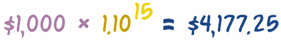... and what if the loan was for 5 years, but the interest rate was only 6%? Here: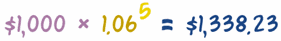Did you see how we just put the 6% into its place like this:... and what if the loan was for 20 years at 8%? ... you work it out!

## Going "Backwards" to Work Out the Present Value

Let's say your goal is to have \$2,000 in 5 Years. You can get 10%, so how much should you start with?

In other words, you know a Future Value, and want to know a Present Value.

We know that multiplying a Present Value (PV) by (1+r)n gives us the Future Value (FV), so we can go backwards by dividing, like this:So the Formula is:

PV = FV(1+r)n

And now we can calculate the answer:

PV = \$2,000(1+0.10)5
= \$2,0001.61051
= \$1,241.84

In other words, \$1,241.84 will grow to \$2,000 if you invest it at 10% for 5 years.

Another Example: How much do you need to invest now, to get \$10,000 in 10 years at 8% interest rate?

PV = \$10,000(1+0.08)10
= \$10,0002.1589
= \$4,631.93

So, \$4,631.93 invested at 8% for 10 Years grows to \$10,000

## Compounding Periods

Compound Interest is not always calculated per year, it could be per month, per day, etc. But if it is not per year it should say so!

Example: you take out a \$1,000 loan for 12 months and it says "1% per month", how much do you pay back?

Just use the Future Value formula with "n" being the number of months:

FV = PV × (1+r)n
= \$1,000 × (1.01)12
= \$1,000 × 1.12683
= \$1,126.83 to pay back

And it is also possible to have yearly interest but with several compoundings within the year, which is called Periodic Compounding.

Example, 6% interest with "monthly compounding" does not mean 6% per month, it means 0.5% per month (6% divided by 12 months), and is worked out like this:

FV = PV × (1+r/n)n
= \$1,000 × (1 + 6%/12)12
= \$1,000 × (1 + 0.5%)12
= \$1,000 × (1.005)12
= \$1,000 × 1.06168...
= \$1,061.68 to pay back

This is equal to a 6.168% (\$1,000 grew to \$1,061.68) for the whole year.

So be careful to understand what is meant!

## APR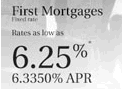but is really 6.335%

Because it is easy for loan ads to be confusing (sometimes on purpose!), the "APR" is often used.

APR means "Annual Percentage Rate": it shows how much you will actually be paying for the year (including compounding, fees, etc).

Here are some examples:

Example 1: "1% per month" actually works out to be 12.683% APR (if no fees).

Example 2: "6% interest with monthly compounding" works out to be 6.168% APR (if no fees).

If you are shopping around, ask for the APR.

## Break Time!

So far we have looked at using (1+r)n to go from a Present Value (PV) to a Future Value (FV) and back again, plus some of the tricky things that can happen to a loan.

Now is a good time to have a break before we look at two more topics:

• How to work out the Interest Rate if you know PV, FV and the Number of Periods.
• How to work out the Number of Periods if you know PV, FV and the Interest Rate

## Working Out The Interest Rate

You can calculate the Interest Rate if you know a Present Value, a Future Value and how many Periods.

Example: you have \$1,000, and want it to grow to \$2,000 in 5 Years, what interest rate do you need?

The formula is:

r = ( FV / PV )1/n - 1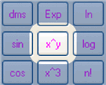Note: the little "1/n" is a Fractional Exponent, first calculate 1/n, then use that as the exponent on your calculator.

For example 20.2 is entered as 2, "x^y", 0, ., 2, =

Now we can "plug in" the values to get the result:

r = ( \$2,000 / \$1,000 )1/5 − 1
= (2)0.2 − 1
= 1.1487 − 1
= 0.1487

And 0.1487 as a percentage is 14.87%,

So you need 14.87% interest rate to turn \$1,000 into \$2,000 in 5 years.

Another Example: What interest rate do you need to turn \$1,000 into \$5,000 in 20 Years?

r = ( \$5,000 / \$1,000 )1/20 − 1
= (5)0.05 − 1
= 1.0838 − 1
= 0.0838

And 0.0838 as a percentage is 8.38%.

So 8.38% will turn \$1,000 into \$5,000 in 20 Years.

## Working Out How Many Periods

You can calculate how many Periods if you know a Future Value, a Present Value and the Interest Rate.

### Example: you want to know how many periods it will take to turn \$1,000 into \$2,000 at 10% interest.

This is the formula (note: it uses the natural logarithm function ln):

n = ln(FV / PV) / ln(1 + r)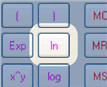The "ln" function should be on a good calculator. You could also use log, just don't mix the two.

Anyway, let's "plug in" the values:

n = ln( \$2,000/\$1,000 ) / ln( 1 + 0.10 )
= ln(2)/ln(1.10)
= 0.69315/0.09531
= 7.27

Magic! It will need 7.27 years to turn \$1,000 into \$2,000 at 10% interest.

### Example: How many years to turn \$1,000 into \$10,000 at 5% interest?

n = ln( \$10,000/\$1,000 ) / ln( 1 + 0.05 )
= ln(10)/ln(1.05)
= 2.3026/0.04879
= 47.19

47 Years! But we are talking about a 10-fold increase, at only 5% interest.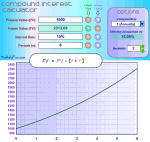## Calculator

I also made a Compound Interest Calculator that uses these formulas.

## Summary

The basic formula for Compound Interest is:

FV = PV (1+r)n

Finds the Future Value, where:

• FV = Future Value,
• PV = Present Value,
• r = Interest Rate (as a decimal value), and
• n = Number of Periods

And by rearranging that formula (see Compound Interest Formula Derivation) we can find any value when we know the other three:

PV = FV(1+r)n

Finds the Present Value when you know a Future Value, the Interest Rate and number of Periods.

r = (FV/PV)(1/n) − 1

Finds the Interest Rate when you know the Present Value, Future Value and number of Periods.

n = ln(FV / PV)ln(1 + r)

Finds the number of Periods when you know the Present Value, Future Value and Interest Rate (note: ln is the logarithm function)

## Annuities

We have now covered what happens to a value as time goes by ... but what if we have a series of values, like regular loan payments or yearly investments? That is covered in the topic of Annuities.